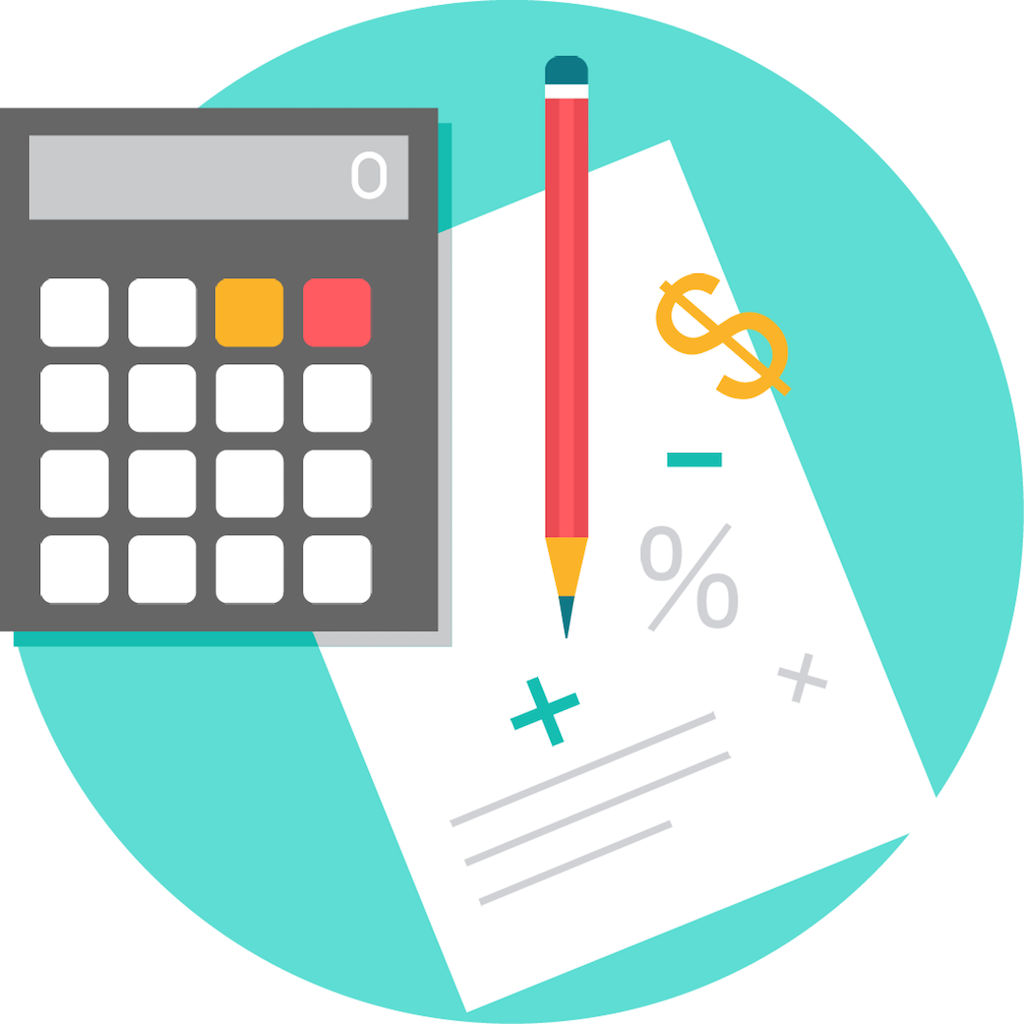#### Current Version : 1.0 Downloads : 586 Size : 14.6 MB

Smart Calculator - Body Calculator and Mathematics Calculator in one app

Body Calculator
BMI (Body Mass Index) - Body Mass Index (BMI) I a measure of body fat based on height and weight that applies to adult men and women. BMI can be used to indicate if you are overweight, obese, underweight or normal. A score below 20 indicates that you are underweight; while score above 25 means you may be overweight. Values shown here falls into following categories:
Underweight
Normal Weight
Overweight
Obese (Class 1) - Moderately obese
Obese (Class 2) - Severely obese
Obese (Class 3) - Very Severely obese
ABF (Army Body Fat) - Army Body Fat Calculator is based on the U.S Army Regulations of Standard Medical Fitness. This calculator will determine your body fat percentage.
Calorie and Carbohydrate Intake - The Carbohydrate Calculator estimates the percentage of carbohydrates you will need each day. The Calorie Calculator estimates the number of calorie a person need to consume each day.
Body Fat - The Body Fat Calculator can be used to estimate your total body fat based on your size.

Math Calculator
Fraction Calculator - Enter numbers of your choice and press “Calculate” button to get result.
Fraction Subtraction
Fraction Multiplication
Fraction Division
Simplify Fraction
Fraction to Decimal
Decimal to Fraction
Percentage Calculator
Calculate Percentage
Percentage Difference
Percentage Increase
Percentage Decrease
Error Find Calculator
Absolute Error
Relative Error
Percent Error
Exponent Calculator -
Root Calculator
Volume Calculator - Vol

ASWDC is established by Department of Computer Engineering where students work on live projects under guidance of staff and industry experts. Students are getting extensive knowledge and industrial experience of cutting edge technologies. ASWDC fills gap between academic curriculum and industry expectation.